## Introduction to Differential Equations

In high school, you studied algebraic equations like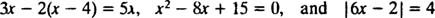The goal here was to solve the equation, which meant to find the value (or values) of the variable that makes the equation true. For example, x = 2 is the solution to the first equation because only when 2 is substituted for the variable x does the equation become an identity (both sides of the equation are identical when and only when x = 2).

In general, each type of algebraic equation had its own particular method of solution; quadratic equations were solved by one method, equations involving absolute values by another, and so on. In each case, an equation was presented (or arose from a word problem), and a certain method was employed to arrive at a solution, a method appropriate for the particular equation at hand.

These same general ideas carry over to differential equations, which are equations involving derivatives. There are different types of differential equations, and each type requires its own particular solution method. The simplest differential equations are those of the form y′ = ƒ( x). For example, consider the differential equationIt says that the derivative of some function y is equal to 2 x. To solve the equation means to determine the unknown (the function y) which will turn the equation into an identity upon substitution. In this case all that is needed to solve the equation is an integration:Thus, the general solution of the differential equation y′ = 2 x is y = x 2 + c, where c is any arbitrary constant. Note that there are actually infinitely many particular solutions, such as y = x 2 + 1, y = x 2 − 7, or y = x 2 + π, since any constant c may be chosen.

Geometrically, the differential equation y′ = 2 x says that at each point ( x, y) on some curve y = y( x), the slope is equal to 2 x. The solution obtained for the differential equation shows that this property is satisfied by any member of the family of curves y = x 2 + c (any only by such curves); see Figure 1.Figure 1

Since these curves were obtained by solving a differential equation—which either explicitly or implicitly involves taking an integral—they are sometimes referred to as integral curves of the differential equation (particularly when these solutions are graphed). If one particular solution or integral curve is desired, the differential equation is appended with one or more supplementary conditions. These additional conditions uniquely specify the value of the arbitrary constant or constants in the general solution. For example, consider the problemThe initial conditiony = 2 when x = 0” is usually abbreviated “ y(0) = 2,” which is read “ y at 0 equals 2.” The combination of a differential equation and an initial condition (also known as a constraint) is called an initial value problem (abbreviated IVP).

For differential equations involving higher derivatives, two or more constraints may be present. If all constraints are given at the same value of the independent variable, then the term IVP still applies. If, however, the constraints are given at different values of the independent variable, the term boundary value problem (BVP) is used instead. For example,but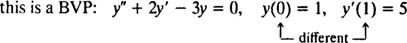To solve an IVP or BVP, first find the general solution of the differential equation and then determine the value(s) of the arbitrary constant(s) from the constraints.

Example 1: Solve the IVP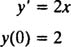As previously noted, the general solution of this differential equation is the family y = x 2 + c. Since the constraint says that y must equal 2 when x is 0,so the solution of this IVP is y = x 2 + 2.

Example 2: Consider the differential equation y″ = 2 y′ − 3 y = 0. Verify that y = c 1 e x + c 2 e −3 x (where c 1 and c 2 are arbitrary constants) is a solution. Given the every solution of this differential equation can be written in the form y = c 1 e x + c 2 e −3 x , solve the IVPTo verify that y = c 1 e x + c 2 e −3 x is a solution of the differential equation, substitute. Since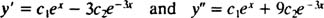once c 1 e x + c 2 e −3 x is substituted for y, the left‐hand side of the differential equation becomesNow, to satisfy the conditions y(0) = 1 and y′(0) = 5, the constants c 1 and c 2 must be chosen so thatandSolving these two equations yields c 1 = 2 and c 2 = − 1. Thus, the particular solution specified by the given IVP is y = 2 e x e −3 x .

The order of a differential equation is the order of the highest derivative that appears in the equation. For example, y′ = 2 x is a first‐order equation, y″ + 2 y′ − 3 y = 0 is a second‐order equation, and y‴ − 7 y′ + 6 y = 12 is a third‐order equation. Note that the general solution of the first‐order equation from Example 1 contained one arbitrary constant, and the general solution of the second‐order equation in Example 2 contained two arbitrary constants. This phenomenon is not coincidental. In most cases, the number of arbitrary constants in the general solution of a differential equation is the same as the order of the equation.

Example 3: Solve the second‐order differential equation y″ = x + cos x.

Integrating both sides of the equation will yield a differential equation for y′: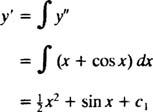Integrating once more will give y: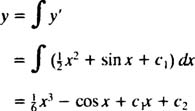where c 1 and c 2 and arbitrary constants. Note that there are two arbitrary constants in the general solution, which you should typically expect for a second‐order equation.

Example 4: For the following IVP, find the solution valid for x > 0: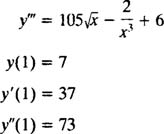The general solution of a third‐order differential equation typically contains three arbitrary constants, so an IVP involving a third‐order differential equation will necessarily have three constraint equations (as is the case here). As in Examples 1 and 3, the given differential equation is of the formwhere y ( n) denotes the nth derivative of the function y. These differential equations are the easiest to solve, since all they require are n successive integrations. Note how the first‐order differential equation in Example 1 was solved with one integration, and the second‐order equation in Example 3 was solved with two integrations. The third‐order differential equation given here will be solved with three successive integrations. Here's the first:The value of this first arbitrary constant ( c 1) can be found by applying the condition y″(1) = 73: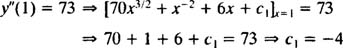Thus, y″ = 70 x 3/2 + x −2 + 6 x − 4.

Now, perform the second integration, which will yield y′:The value of this arbitrary constant ( c 2) can be found by applying the constraint y′(1) = 37: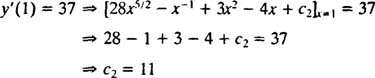Therefore, y′ = 28 x 5/2x −1 + 3 x 2 − 4 x + 11. Integrating once more will give the solution y:The value of this arbitrary constant ( c 3) can be found by applying the condition y(1) = 7:Thus, the solution is y = 8 x 7/2 − In x + x 3 − 2 x 2 + 11 x − 11.

• The given differential equation makes sense only for x > 0 (note theand 2/ x 3 terms). To respect this restriction, the problem states the domain of the equation and its solution [that is, the set of values of the variable(s) where the equation and solution are valid] as x > 0. Always be aware of the domain of the solution.
• Although the integral of x −1 is usually written In | x|, the absolute value sign is not needed here, since the domain of the solution is x > 0, and | x| = x for any x > 0.
• Constrast the methods used to evaluate the arbitrary constants in Examples 2 and 4. In Example 2, the constraints were applied all at once at the end. In Example 4, however, the constants were evaluated one at a time as the solution progressed. Both methods are valid, and each particular problem (and your preference) will suggest which to use.

Example 5: Find the differential equation for the family of curves x 2 + y 2 = c 2 (in the xy plane), where c is an arbitrary constant.

This problem is a reversal of sorts. Typically, you're given a differential equation and asked to find its family of solutions. Here, on the other hand, the general solution is given, and an expression for its defining differential equation is desired. Differentiating both sides of the equation (with respect to x) gives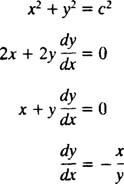This differential equation can also be expressed in another form, one that will arise quite often. By “cross multiplying,” the differential equation directly above becomeswhich is then normally written with both differentials (the dx and the dy) together on one side: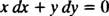Either y′ = − x/ y or x dx + y dy = 0 would be an acceptable way of writing the differential equation that defines the given family (of circles) x 2 + y 2 = c 2.

Example 6: Verify that the equation y = In ( x/y) is an implicit solution of the IVPFirst note that it is not always possible to express a solution in the form “ y = some function of x.” Sometimes when a differential equation is solved, the solution is most naturally expressed with y's (the dependent variable) on both sides of the equation, as in y = In( x/y). Such a solution is called an implicit solution, as opposed to an explicit solution, which has y all by itself on one side of the equation and a function of x only on the right (as in y = x 2 + 2, for example). Implicit solutions are perfectly acceptable (in some cases, necessary) as long as the equation actually defines y as a function of x (even if an explicit formula for this function is not or cannot be found). However, explicit solutions are preferable when available.

Perhaps the simplest way to verify this implicit solution is to follow the procedure of Example 5: Find the differential equation for the solution y = In( x/y). To simplify the work, first rewrite In( x/y) as In x − In y: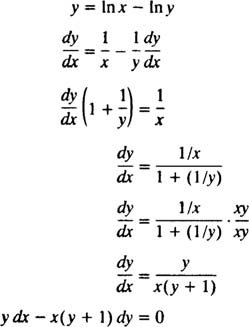Therefore, the differential equation given in the statement of the problem is indeed correct. The initial condition is also satisfied, since 1 = In( e/1) implies y( e) = 1 satisfies y = In( x/y).

Example 7: Discuss the solution to each of the differential equations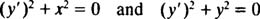The first differential equation has no solution, since non realvalued function y = y( x) can satisfy ( y′) 2 = − x 2 (because squares of real‐valued functions can't be negative).

The second differential equation states that the sum of two squares is equal to 0, so both y′ and y must be identically 0. This equation does have a solution, but it is only the constant function y ≡ 0. Note that this differential equation illustrates an exception to the general rule stating that the number of arbitrary constants in the general solution of a differential equation is the same as the order of the equation. Although ( y′) 2 + y 2 is a first‐order equation, its general solution y ≡ 0 contains no arbitrary constants at all.

One final note: Since there are two major categories of derivatives, ordinary derivatives like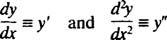and partial derivatives such as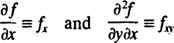there are two major categories of differential equations. Ordinary differential equations (ODEs) involve ordinary derivatives, while partial differential equations (PDEs), such as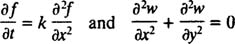involve partial derivatives.